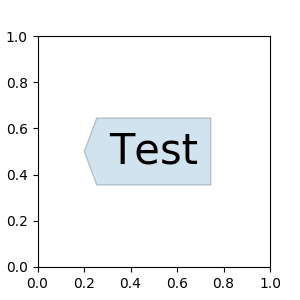# Custom Boxstyle01¶```from matplotlib.path import Path

def custom_box_style(x0, y0, width, height, mutation_size, mutation_aspect=1):
"""
Given the location and size of the box, return the path of
the box around it.

- *x0*, *y0*, *width*, *height* : location and size of the box
- *mutation_size* : a reference scale for the mutation.
- *aspect_ratio* : aspect-ration for the mutation.
"""

# note that we are ignoring mutation_aspect. This is okay in general.

width = width + 2 * pad
height = height + 2 * pad

# boundary of the padded box
x1, y1 = x0 + width, y0 + height

cp = [(x0, y0),
(x1, y0), (x1, y1), (x0, y1),
(x0, y0)]

com = [Path.MOVETO,
Path.LINETO, Path.LINETO, Path.LINETO,
Path.LINETO, Path.LINETO,
Path.CLOSEPOLY]

path = Path(cp, com)

return path

import matplotlib.pyplot as plt

fig, ax = plt.subplots(figsize=(3, 3))
ax.text(0.5, 0.5, "Test", size=30, va="center", ha="center",
bbox=dict(boxstyle=custom_box_style, alpha=0.2))

plt.show()
```

Keywords: matplotlib code example, codex, python plot, pyplot Gallery generated by Sphinx-Gallery